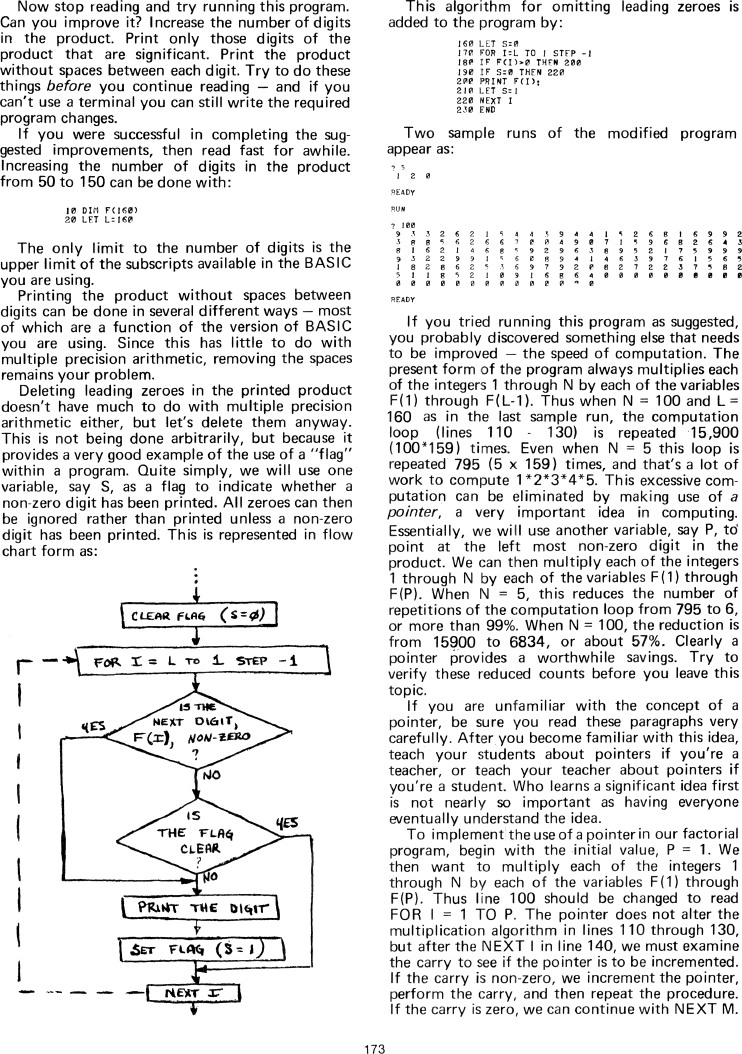The Best of Creative Computing Volume 1 (published 1976)

 `Page 173 << PREVIOUS >> NEXT Jump to page: Go to contents Go to thumbnails`

Computing Factorials Accurately```Now stop reading and try running this program. Can you improve it? Increase the
number of digits in the product. Print only those digits of the product that are
significant. Print the product without spaces between each digit. Try to do
these things before you continue reading - and if you can't use a terminal you
can still write the required program changes.

If you were successful in completing the suggested improvements, then read fast
for awhile. Increasing the number of digits in the product from 50 to 150 can be
done with:

10 DIM F(160)
20 LET L=160

The only limit to the number of digits is the upper limit of the subscripts
available in the BASIC you are using.

Printing the product without spaces between digits can be done in several
different ways - most of which are a function of the version of BASIC you are
using. Since this has little to do with multiple precision arithmetic, removing

Deleting leading zeroes in the printed product doesn't have much to do with
multiple precision arithmetic either, but let's delete them anyway.This is not
being done arbitrarily, but because it provides a very good example of the use
of a "flag" within a program. Quite simply, we will use one variable, say S, as
a flag to indicate whether a non-zero digit has been printed. All zeroes can
then be ignored rather than printed unless a non-zerodigit has been printed.
This is represented in flow
chart form as:

[image]

This algorithm for omitting leading zeroes is

160 LET S=0
|70 FOR I=L T0 1 STFP -l
180 IF F(I)>0 THEN 200
190 IF S=0 THEN 220
200 PRINT F(I):

210 LET S=1
220 NEXT 1
230 END

Two sample runs of the modified program
appear as:

[image]

If you tried running this program as suggested, you probably discovered
something else that needs to be improved - the speed of computation. The present
form of the program always multiplies each
of the integers 1 through N by each of the variables F(1) through F(L-1). Thus
when N = 100 and L= 160 as in the last sample run, the computation loop (lines
110 - 130) is repeated 15,900 (100*159) times. Even when N = 5 this loop is
repeated 795 (5 x 159) times, and that's a lot of work to compute 1*2*3*4*5.
This excessive computation can be eliminated by making use of a pointer, a very
important idea in computing. Essentially, we will use another variable, say P,
to point at the left most non-zero digit in the product. We can then multiply
each of the integers 1 through N by each of the variables F(1) through F(P).
When N = 5, this reduces the number of repetitions of the computation loop from
795 to 6, or more than 99%. When N = 100, the reduction is from 15900 to 6834,
or about 57%. Clearly a pointer provides a worthwhile savings. Try to verify
these reduced counts before you leave this topic.

If you are unfamiliar with the concept of a pointer, be sure you read these
paragraphs very carefully. After you become familiar with this idea, teach your
pointers if you're a student. Who learns a significant idea first is not nearly
so important as having everyone eventually understand the idea.

To implement the use of a pointer in our factorial program, begin with the
initial value, P = 1. We then want to multiply each of the integers 1 through N
by each of the variables F(1) through F(P). Thus line 100 should be changed to
read FOR I = 1 TO P. The pointer does not alter the multiplication algorithm in
lines 110 through 130, but after the NEXT l in line 140, we must examine the
carry to see if the pointer is to be incremented. If the carry is non-zero, we
increment the pointer, perform the carry, and then repeat the procedure. If the
carry is zero, we can continue with NEXT M.Page 173        << PREVIOUS        >> NEXT        Jump to page:                Go to contents        Go to thumbnails

<!--